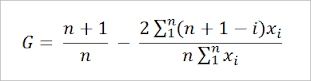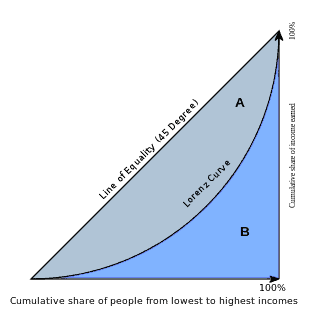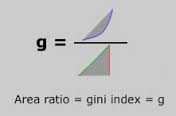Gini index definition

Gini index definition – fundamental analysis
Gini index serves for defining the economic difference between socially difference among the residents. The index is ranged between the values of 0 and 1, where the zero means that residents are all equal and 1 means that there is a huge gap between them.
The index got its name after Gini Corrado who developed it. He was an Italian statistician who worked in the beginning of the twentieth century.

Gini index calculation – gini index formula:How to calculate gini index:
We can get Gini index from Lorenz curve as G = A / (A + B) where A and B are :The distribution of income is commonly mistaken with the wealth of the residents, but it is not about that, it is rather about the difference of the distribution of it.
The accuracy of the Gini index heavily depends on the accuracy of information about the GDP. Since the countries in economical progress usually give inaccurate data about their GDP. The Gini index is often not as accurate.

Gini index example:
Draw Gini index:

Calculate:

Below you can see how to calculate Gini index in Excel – calculating gini coefficient using excel and measuring the gini coefficient in excel.

If you know more how we can compute gini excel – please send comment below.